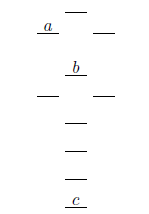# Problem: Consider the molecular orbital diagram for a 2nd row diatomic molecule (X 2) shown below. The labels a, b, and c are placed in three of the molecular orbitals. What are the names of these three orbitals? 1. σ*2p, π2p, σ*1s 2. π*2p, σ*2p, σ1s 3. σ*2p, π2p, σ*2s 4. π*2p, σ2p, σ1s 5. π*2p, σ2p, σ*2s

###### Problem Details

Consider the molecular orbital diagram for a 2nd row diatomic molecule (X 2) shown below. The labels a, b, and c are placed in three of the molecular orbitals. What are the names of these three orbitals?

1. σ*2p, π2p, σ*1s

2. π*2p, σ*2p, σ1s

3. σ*2p, π2p, σ*2s

4. π*2p, σ2p, σ1s

5. π*2p, σ2p, σ*2sWhat scientific concept do you need to know in order to solve this problem?

Our tutors have indicated that to solve this problem you will need to apply the Homonuclear Diatomic Molecules concept. If you need more Homonuclear Diatomic Molecules practice, you can also practice Homonuclear Diatomic Molecules practice problems.

What is the difficulty of this problem?

Our tutors rated the difficulty ofConsider the molecular orbital diagram for a 2nd row diatomi...as high difficulty.

How long does this problem take to solve?

Our expert Chemistry tutor, Dasha took 1 minute and 54 seconds to solve this problem. You can follow their steps in the video explanation above.

What professor is this problem relevant for?

Based on our data, we think this problem is relevant for Professor Shear's class at TEXAS.